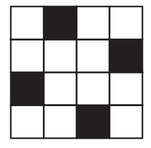Q

# Copy the figure given here. Take any one diagonal as a line of symmetry and shade a few more squares to make the figure symmetric about a diagonal. Is there more than one way to do that? Will the figure be symmetric about both the diagonals ?

5.    Copy the figure given here.
Take any one diagonal as a line of symmetry and shade a few more squares to make the figure symmetric about a diagonal. Is there more than one way to do that? Will the figure be symmetric about both the diagonals?Views

The figure with symmetry may be as shown :Yes,there more than one way .

Yes,the figure be symmetric about both the   diagonals

Exams
Articles
Questions